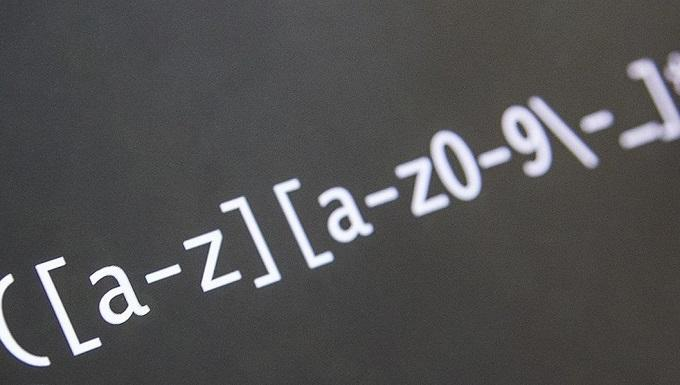## 1. 引言

• Lookbehind assertions - 后行断言
• Named capture groups - 命名捕获组
• s (dotAll) Flag - . 匹配任意字符
• Unicode property escapes - Unicode 属性转义

## 2.1. Lookbehind assertions

``````const re = /Item(?= 10)/;

console.log(re.exec("Item"));
// → null

console.log(re.exec("Item5"));
// → null

console.log(re.exec("Item 5"));
// → null

console.log(re.exec("Item 10"));
// → ["Item", index: 0, input: "Item 10", groups: undefined]``````

``````const re = /Red(?!head)/;

// → null

console.log(re.exec("Redberry"));
// → ["Red", index: 0, input: "Redberry", groups: undefined]

console.log(re.exec("Redjay"));
// → ["Red", index: 0, input: "Redjay", groups: undefined]

console.log(re.exec("Red"));
// → ["Red", index: 0, input: "Red", groups: undefined]``````

``````const re = /(?<=€)\d+(\.\d*)?/;

console.log(re.exec("199"));
// → null

console.log(re.exec("\$199"));
// → null

console.log(re.exec("€199"));
// → ["199", undefined, index: 1, input: "€199", groups: undefined]``````

``````const re = /(?<!\d{3}) meters/;

console.log(re.exec("10 meters"));
// → [" meters", index: 2, input: "10 meters", groups: undefined]

console.log(re.exec("100 meters"));
// → null``````

``````const re = /(?<=\d{2})(?<!35) meters/;

console.log(re.exec("35 meters"));
// → null

console.log(re.exec("meters"));
// → null

console.log(re.exec("4 meters"));
// → null

console.log(re.exec("14 meters"));
// → ["meters", index: 2, input: "14 meters", groups: undefined]``````

## 2.2. Named Capture Groups

``````const re = /(?<year>\d{4})-(?<month>\d{2})-(?<day>\d{2})/;
const [match, year, month, day] = re.exec("2020-03-04");

console.log(match); // → 2020-03-04
console.log(year); // → 2020
console.log(month); // → 03
console.log(day); // → 04``````

``````console.log(/(\w\w)\1/.test("abab")); // → true

// if the last two letters are not the same
// as the first two, the match will fail
console.log(/(\w\w)\1/.test("abcd")); // → false``````

``````const re = /\b(?<dup>\w+)\s+\k<dup>\b/;

const match = re.exec("I'm not lazy, I'm on on energy saving mode");

console.log(match.index); // → 18
console.log(match); // → on on``````

## 2.3. s (dotAll) Flag

``````console.log(/./s.test("\n")); // → true
console.log(/./s.test("\r")); // → true``````

## 2.4. Unicode Property Escapes

u 修饰符可以识别所有大于 0xFFFF 的 Unicode 字符。
``````const regex = /^\p{Number}+\$/u;
regex.test("²³¹¼½¾"); // true
regex.test("㉛㉜㉝"); // true
regex.test("ⅠⅡⅢⅣⅤⅥⅦⅧⅨⅩⅪⅫ"); // true``````

`\p{Alphabetic}` 可以匹配所有 Alphabetic 元素，包括汉字、字母等：

``````const str = "漢";

console.log(/\p{Alphabetic}/u.test(str)); // → true

// the \w shorthand cannot match 漢
console.log(/\w/u.test(str)); // → false``````

## 3.1. RegExp 构造函数优化

``new RegExp(/book(?=s)/giu, "iu");``

## 3.2. 字符串的正则方法

`String.prototype.match` 指向 `RegExp.prototype[Symbol.match]`

``````"abc".match(/abc/g) /
// 内部执行时，等价于
abc /
g[Symbol.match]("abc");``````

## 3.3. u 修饰符

`u` 修饰符的含义为 “Unicode 模式”，用来正确处理大于 `\uFFFF` 的 Unicode 字符。

• 点字符原本支持单字符，但在 `u` 模式下，可以匹配大于 `0xFFFF` 的 Unicode 字符。
• `\u{61}` 含义由匹配 61 个 `u` 改编为匹配 Unicode 编码为 61 号的字母 `a`
• 可以正确识别非单字符 Unicode 字符的量词匹配。
• `\S` 可以正确识别 Unicode 字符。
• `u` 模式下，`[a-z]` 还能识别 Unicode 编码不同，但是字型很近的字母，比如 `\u212A` 表示的另一个 `K`

## 3.4. y 修饰符

`y` 修饰符是 “粘连”（sticky）修饰符。

`y` 类似 `g` 修饰符，都是全局匹配，也就是从上次成功匹配位置开始，继续匹配。`y` 的区别是，必须是上一次匹配成功后的下一个位置就立即匹配才算成功。

``/a+/g.exec("aaa_aa_a"); // ["aaa"]``

## 3.5. flags

``````const regex = /[a-z]*/gu;

regex.flags; // 'gu'``````

## 4. 总结

ES2018 引入的特性还太新，单在对 ES6 特性的使用应该和对 ES3 一样熟练。